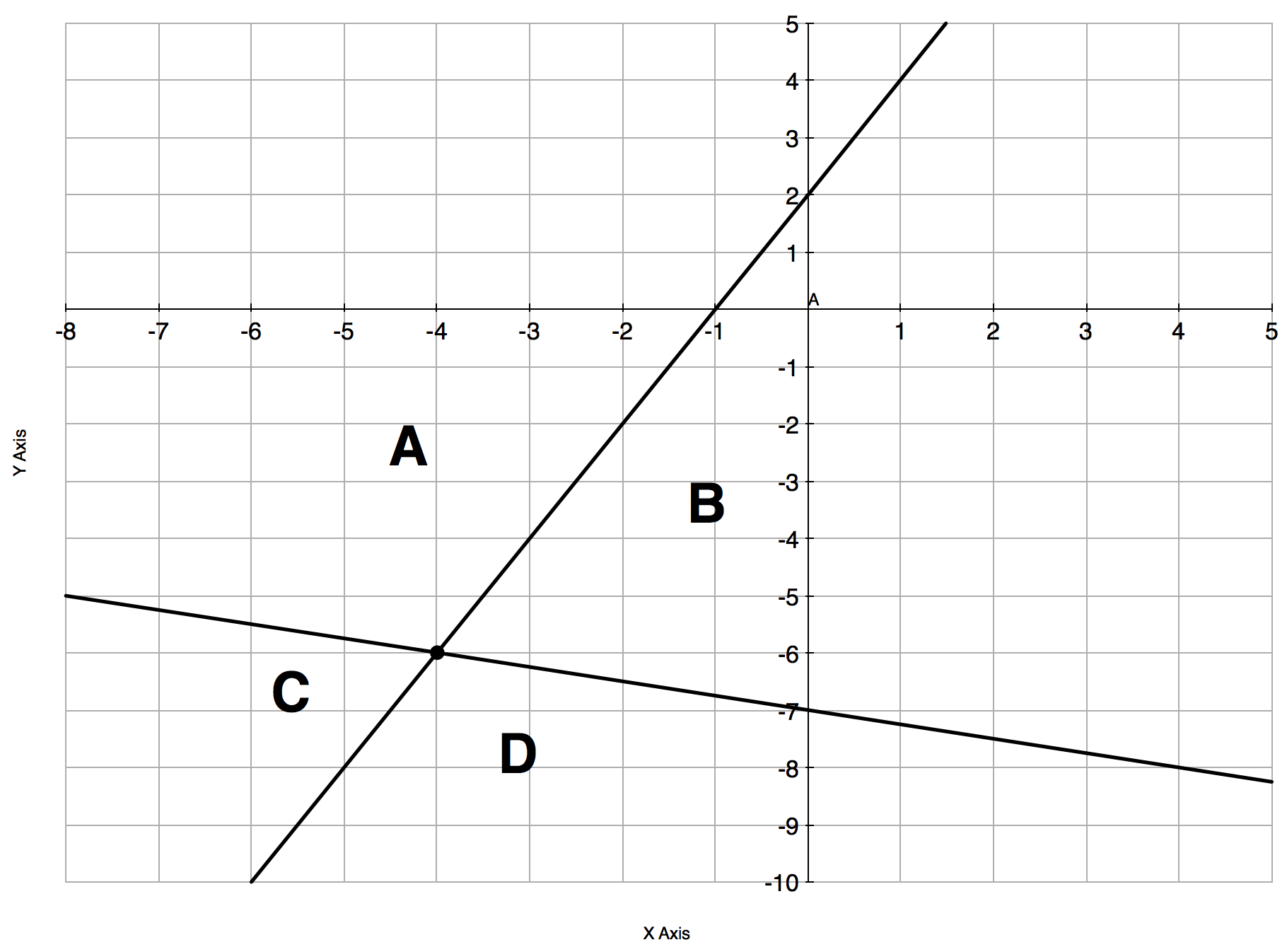# Linear Equations, Inequalities and Systems

## Objective

Identify solutions to systems of inequalities graphically. Write systems of inequalities from graphs and word problems.

## Common Core Standards

### Core Standards

?

• A.CED.A.3 — Represent constraints by equations or inequalities, and by systems of equations and/or inequalities, and interpret solutions as viable or nonviable options in a modeling context. For example, represent inequalities describing nutritional and cost constraints on combinations of different foods.

• A.REI.D.12 — Graph the solutions to a linear inequality in two variables as a half-plane (excluding the boundary in the case of a strict inequality), and graph the solution set to a system of linear inequalities in two variables as the intersection of the corresponding half-planes.

?

• 8.EE.C.8

## Criteria for Success

?

### Problem 1

Some treasure has been buried at a point ${(x,y)}$ on the grid, where $x$ and $y$ are whole numbers.

Clue 1: $x> 2$
Clue 2: $x+y< 8$
Clue 3: $2y-x\geq 0$

Which of the following points could be a possible location for the treasure?

(3,2)     (2,3)     (5,3)     (3,5)     (4,3)     (5,2)

### Problem 2

Write a system of linear inequalities that only has the region named as part of the solution set.## Problem Set

?

The following resources include problems and activities aligned to the objective of the lesson that can be used to create your own problem set.

• Include problems where there is no solution for the system of inequalities as well as all solutions for the system of inequalities.